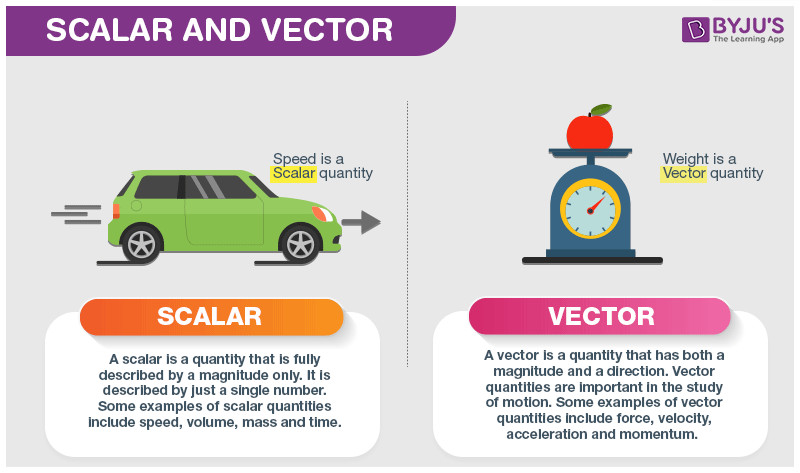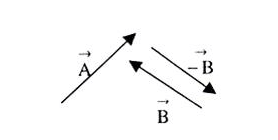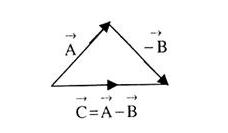# Scalar And Vector

Mathematics and Science were invented by humans to understand and describe the world around us. A lot of mathematical quantities are used in Physics to explain the concepts clearly. A few examples of these include force, speed, velocity and work. These quantities are often described as being a scalar or a vector quantity. Scalars and vectors are differentiated depending on their definition. A scalar quantity is defined as the physical quantity that has only magnitude, for example, mass and electric charge. On the other hand, a vector quantity is defined as the physical quantity that has both magnitude as well as direction like force and weight. The other way of differentiating these two quantities is by using a notation. In this article, let us try to learn what is a vector and a scalar quantity.## What Is Scalar Quantity?

Scalar quantity is defined as the physical quantity with magnitude and no direction.

Some physical quantities can be described just by their numerical value (with their respective units) without directions (they don’t have any direction). The addition of these physical quantities follows the simple rules of the algebra. Here, only their magnitudes are added.

### Examples of Scalar Quantities

There are plenty of scalar quantity examples, some of the common examples are:

• Mass
• Speed
• Distance
• Time
• Area
• Volume
• Density
• Temperature

## What is a Vector Quantity?

A vector quantity is defined as the physical quantity that has both direction as well as magnitude.

A vector with the value of magnitude equal to one and direction is called unit vector represented by a lowercase alphabet with a “hat” circumflex. That is “û“.

### Examples of Vector Quantities

Vector quantity examples are many, some of them are given below:

• Linear momentum
• Acceleration
• Displacement
• Momentum
• Angular velocity
• Force
• Electric field
• Polarization## Difference Between Scalar and Vector

The difference between Scalar and Vector is crucial to understand in physics learning. Below are a few differences for better understanding.

Vector Scalar A physical quantity with both the magnitude and direction. A physical quantity with only magnitude. A number (magnitude), direction using unit cap or arrow at the top and unit. A number (magnitude) and Unit Quantity symbol in bold and an arrow sign above Quantity symbol Yes No Velocity and Acceleration Mass and Temperature

After understanding what is a vector, let’s learn vector addition and subtraction. The addition and subtraction of vector quantities does not follow the simple arithmetic rules. A special set of rules are followed for the addition and subtraction of vectors. Following are some points to be noted while adding vectors:

• Addition of vectors means finding the resultant of a number of vectors acting on a body.
• The component vectors whose resultant is to be calculated are independent of each other. Each vector acts as if the other vectors were absent.
• Vectors can be added geometrically but not algebraically.
• Vector addition is commutative in nature, i.e., $$\underset{A}{\rightarrow}+\underset{B}{\rightarrow}=\underset{B}{\rightarrow}+\underset{A}{\rightarrow}$$

Now, talking about vector subtraction, it is the same as adding the negative of the vector to be subtracted. TO better understand, let us look at the example given below.
Let us consider two vectors $$\underset{A}{\rightarrow}$$ and $$\underset{B}{\rightarrow}$$ as shown in the figure below. We required to subtract $$\underset{B}{\rightarrow}$$ from $$\underset{A}{\rightarrow}$$. It is just the same as adding $$\underset{-B}{\rightarrow}$$ and $$\underset{A}{\rightarrow}$$. The resultant is shown in the figure below### Vector Notation

For vector quantity usually, an arrow is used on the top like $$\underset{v}{\rightarrow}$$ which represents the vector value of the velocity and also explains that the quantity has both magnitudes as well as direction.

Following is the table explaining other related concepts:

## Scalar and Vector Quantities Problems with Solutions

Q1: Given below is a list of quantities. Categorize each quantity as being either a vector or a scalar.

 20 degrees Celsius 5 mi., North 256 bytes 5 m 30 m/sec, East 4000 Calories

 20 degrees Celsius Scalar 5 mi., North Vector 256 bytes Scalar 5 m Scalar 30 m/sec, East Vector 4000 Calories Scalar

Q2: Ashwin walks 10 m north, 12 m east, 3 m west and 5 m south and then stops to drink water. What is the magnitude of his displacement from his original point?

Answer: We know that displacement is a vector quantity, hence the direction Ashwin walks will either be positive or negative along an axis.

Now, to find the total distance travelled along the y-axis, let us consider the movement towards the north to be positive and the movement towards the south to be negative.

$$\sum y=10\,m-5\,m=5\,m$$

He moved a net of 5 meters to the north along the y-axis.

Similarly, let us consider his movement towards the east to be positive and the movement towards the west to be negative.

$$\sum y=-3\,m+12\,m=9\,m$$

He moved a net of 9 m to the east.

Using Pythagoras theorem, the resultant displacement can be found as follows:

$$D^2=(\sum x^2)+(\sum y^2)$$

Substituting the values, we get

$$D^2=(9^2)+(5^2)$$ $$D^2=(106)^2$$ $$\sqrt{D^2}=\sqrt{(106)^2}$$ $$D=10.30\,m$$

Q3. What is the magnitude of a unit vector?

Answer: The magnitude of a unit vector is unity. A unit vector has no units or dimensions.

To know more about what is a vector, vector addition and subtraction, stay tuned with BYJU’S. Also, register to “BYJU’S – The Learning App” for loads of interactive, engaging Physics-related videos and an unlimited academic assist.

Test your Knowledge on Scalars And Vectors

1. Why Kinetic Energy is a scalar quantity while velocity is a vector quantity?

1. Kinetic energy is a scalar quantity because it doesn’t have a direction, unlike velocity. The kinetic energy of an object is completely described by magnitude alone.

its velocity square and the dot product of two vectors is a scalar quantity .hence,
kinetic energy isa scalar quantity

2. Pratibha Menda

How weight is vector quantity

1. The gravitational acceleration is a vector quantity which has magnitude and direction. As a result, the weight of the object is a vector quantity because of its gravitational acceleration.

3. Kaif Sufi

Why do we need vector and scalar?

1. Many quantities in Physics are vectors and when we try to add two vectors without considering their directions we usually end up with wrong results. Hence, vectors are important for accurate results.

Even though scalars signify just the magnitude, they are used all the time in Physics.

4. Sonu mishr khan patel singh

What is use in daily life of scalar and vector quantities